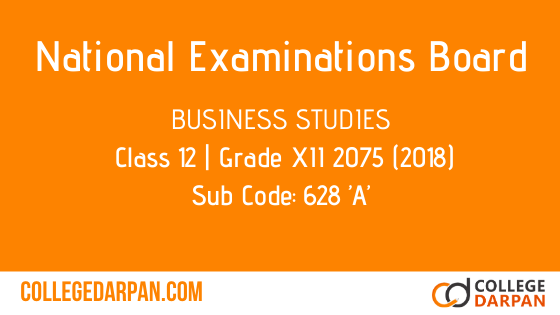Subject Code: 628 ’A’

2075 (2018)

Candidates are required to give their answers in their own words as far as practicable. The figures in the margin indicate full marks.

Time – 3 hrs

Full Marks – 100

Pass Marks – 35 (Only for partial students)

(Group ‘A’)

(Attempt any Eight questions) [8×8=64]

1. (Explain management as a science and an art.) [4+4]

2. (What is planning? Explain the importance of planning in an organization.)  [2+6=8]

3. (Give the concept of management decision and explain the steps involved in decision making process.)  [2+6=8]

4. (Explain the components of direction?) 

5. (Describe about Maslow’s need hierarchy theory of motivation.) 

7. (Give the concept of supervision and explain the factors affecting supervision. [2+6=8]

8. (Explain the different types of communication.) 

9. (What is co-ordination? Explain the techniques of co-ordination.)  [2+6=8]

10. (Give the concept of control and explain it’s processes.)  [2+6=8]

(Or)

(Explain the importance of participative management in organizations.) 

(Group ‘B’)

(Attempt any two questions)    [2×18=36]

11. (Explain the administrative management principles introduced by Henry Fayol.)  

12. (Clarify about delegation of authority and show the difference between delegation of authority and decentralization.)
[6+12=18]

13. (What is insurance? Describe the fundamental principles of insurance contract.)
[6+12=18]